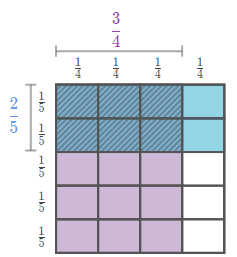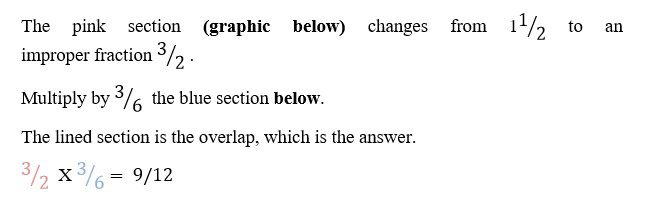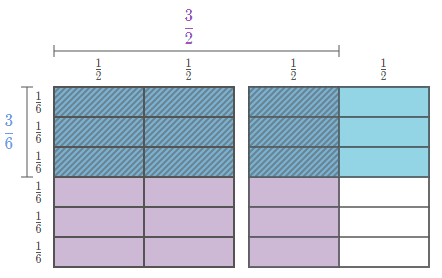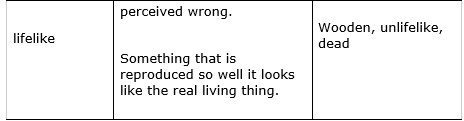• Buckinghamshire Tutors

# Expertly-chosen and Created Materials for 11+ with the GL Exam Board – Week 8.Welcome to week 8. For newcomers, this blog is free for all users, all the clips, questions, and advice have been carefully chosen by me, a highly experienced 11+ tutor. This is not only suitable for children in Buckinghamshire, but for children in any area that uses the GL exam board.

## Multiplying fractionsWhen we need to find a fraction OF a number, we multiply it to get the answer.

That may seem odd, but if we multiply a number by a fraction the number will always get smaller. That is because you are not multiplying by whole numbers, but by less than 1, so of course it will get smaller.

• First, simplify each fraction, if possible
• Second, multiply the top fractions (remember the top number on the top is called the numerator)
• Third, multiply the bottom fractions (the fraction on the bottom is called the denominator)
• Fourth, simplify the answer, if possible.

To find a fraction of a whole number or a fraction of a fraction, we multiply the fractions as in the video below.http://www.bbc.co.uk/guides/z8fyv4j#z33bg82Tip: This isn’t explained in the video below, but you need to know it when answering the questions at the end of the video; when multiplying mixed numbers, make the mixed number into an improper fraction.Remember: ten a day, so practise a different section every day:https://uk.ixl.com/math/year-5/multiply-unit-fractions-by-whole-numbers-using-number-lines

https://uk.ixl.com/math/year-5/multiply-unit-fractions-by-whole-numbers-using-models

https://uk.ixl.com/math/year-5/multiples-of-fractions

https://uk.ixl.com/math/year-5/multiply-unit-fractions-and-whole-numbers-sorting

https://uk.ixl.com/math/year-5/multiply-unit-fractions-by-whole-numbers-word-problems

https://uk.ixl.com/math/year-5/multiply-fractions-by-whole-numbers-using-number-lines

https://uk.ixl.com/math/year-5/multiply-fractions-by-whole-numbers-using-models

https://uk.ixl.com/math/year-5/multiply-fractions-by-whole-numbers-word-problems

https://uk.ixl.com/math/year-5/multiply-fractions-by-whole-numbers-ii

https://uk.ixl.com/math/year-5/multiply-fractions-by-whole-numbers-word-problems

## Use a Rule to Make a WordHere are some for you to do:

(I’ve put the numbers in the first one for you....)

1. frog (long) lend ware (- - - -) tied
2. home (east ) cast nest (- - - -) woes
3. meal (meat) fast when (- - - -) drat
4. miss ( mown) down that (- - - -) core
5. busy (yell) fell went (- - - -) make
6. lock (peck ) dupe whir (- - - -) lost

Sometimes a letter could have come from two places. This is where it gets complicated and you need a system.There are two places the E could have come from. The E’s do not have to remain in the same position, so E could have come from position 2 word 1, or position 3 word 2.

But you look for the word and see: wart. That is your answer.

More practice: (I have put the first set of numbers in for you.)

1. mist (dust) used true ( - - - -) lamb

- - 34 23-1 - - - - - - - -

The letters in position 3 could have come from two places.

So we h ave to reflect that in the second set.

But only one combination makes a word: - - - -

1. slug (shut) huts sham (- - - - ) calm
1. bred (bead) lean lord ( - - - - ) pant

10) bear (near) nice like ( - - - - ) hole

11) shrew (white) hints sharp (- - - - -) apres

Answers to Use a Rule to Make a Word

### Vocabulary

This week, the vocabulary work is a bit different. We are focusing on compound words. These are words that have been used together so often that they became one word, with an identity all of its own, a sort of baby word – isn’t that sweet?Next week - Non-Verbal Reasoning, Verbal Reasoning, Vocab